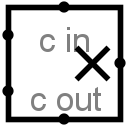#Multiplizierer

 Bibliothek: Arithmetic Eingeführt: 2.0 Beta 20 Aussehen:## Verhalten

This component multiplies two values coming in via the west inputs and outputs the product on the east output. The component is designed so that it can be cascaded with other multipliers to multiply a multiplicand with more bits than is possible with a single multiplier: The carry-in input provides a multi-bit value to be added into the product (if it is specified), and a carry-out output provides the upper half of the product result, which can be fed into another multiplier.

If the multiplicand, the multiplier, or the carry-in input contain some floating bits or some error bits, then the component will perform a partial multiplication. That is, it will compute as many low-order bits as possible. But above the floating or error bit, the result will have floating or error bits. Note that if the carry-in input is completely floating, then it will be assumed to be all-zeroes.

## Pins

West edge, north end (input, bit width matches Data Bits attribute)
The multiplicand (that is, the first of the two numbers to multiply).
West edge, south end (input, bit width matches Data Bits attribute)
The multiplier (that is, the second of the two numbers to multiply).
North edge, labeled c in (input, bit width matches Data Bits attribute)
A carry value to add into the product. If all bits of the value are unknown (i.e., floating), then they are assumed to be 0.
East edge (output, bit width matches Data Bits attribute)
The lower dataBits bits of the product of the two values coming in the west edge, plus the cin value.
South edge, labeled c out (output, bit width matches Data Bits attribute)
The upper dataBits bits of the product.

## Attribute

When the component is selected or being added, Alt-0 through Alt-9 alter its Data Bits attribute.

Data Bits
The bit width of the values to be multiplied and of the result.

Keines.

## Verhalten des Textwerkzeugs

Keines.

Zurück zur Bibliotheksreferenz